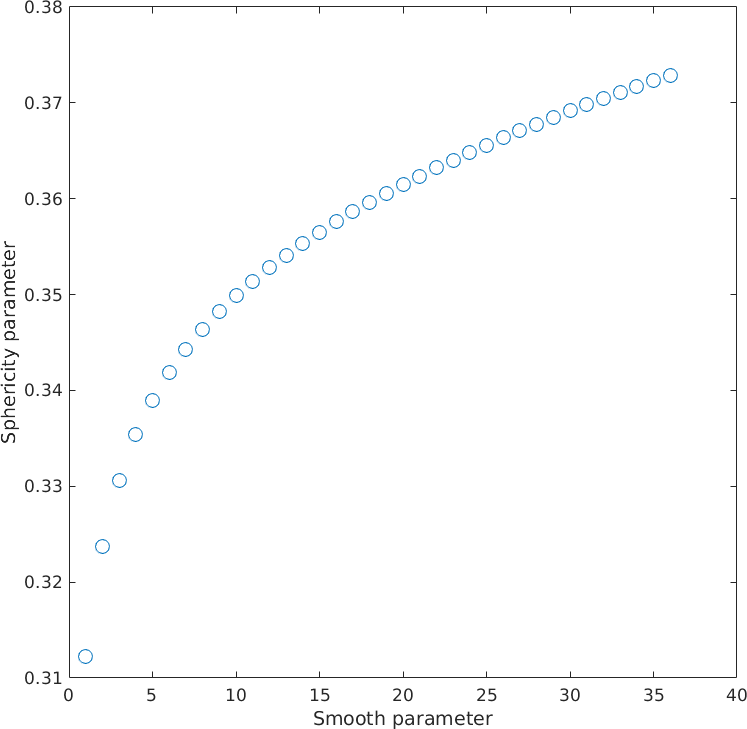Grain Boundary Statistics in Deformed Ice edit page author: Sheng Fan, Department of Geology, University of Otago, Dunedin, New Zealand

## The sphericity as measure for grain boundary irregularity

Deformed polycrystalline materials such as ice often contain large grains interlocking with smaller grains, with many irregular grain boundaries. Boundary irregularity is hard to judge by visual inspection and it is better to use quantitative measures of boundary irregularity to infer processes across different deformation conditions. Here, we quantify the irregularity of each grainÿs boundary by introducing a sphericity parameter $$\Psi$$ which is calculated in 2-D from grain area A, grain boundary perimeter P, and area equivalent grain radius R by the formula

$\Psi = \frac{A}{P \cdot R}$

The grain sphericity $$\Psi$$ is a useful indicator for grain boundary irregularity because it measures how closely a grainÿs boundary resembles the circumference of a perfect circle. It decreases from $$\Psi = 0.5$$, where the grain has a perfect circular shape, to $$\Psi = 0$$ where the grain boundary is infinitely irregular. The statistics of grain boundary sphericity can be used to segregate recrystallised grains from remnant original grains (please refer to the paper for more details).

## Data

The EBSD data set used in this demonstration (PIL185.ctf) is available from https://doi.org/10.6084/m9.figshare.13456550. The EBSD data were collected with a step size of 5 µm and representeds an ice sample deformed at -20°C to 12 percent axial strain. Let's import the data and reconstruct some grains.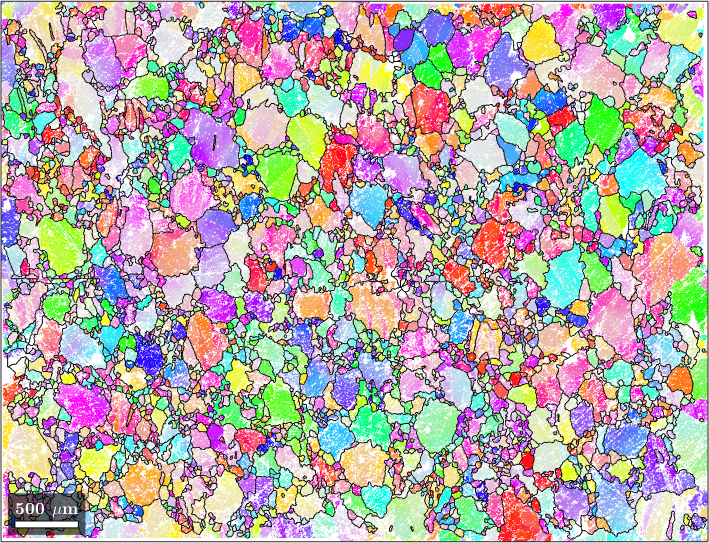## Computation of the Sphericity

Next, let's calculate and plot the sphericity parameter of each ice grain by making use of the functions grains.area, grains.perimeter and grains.equivalentRadius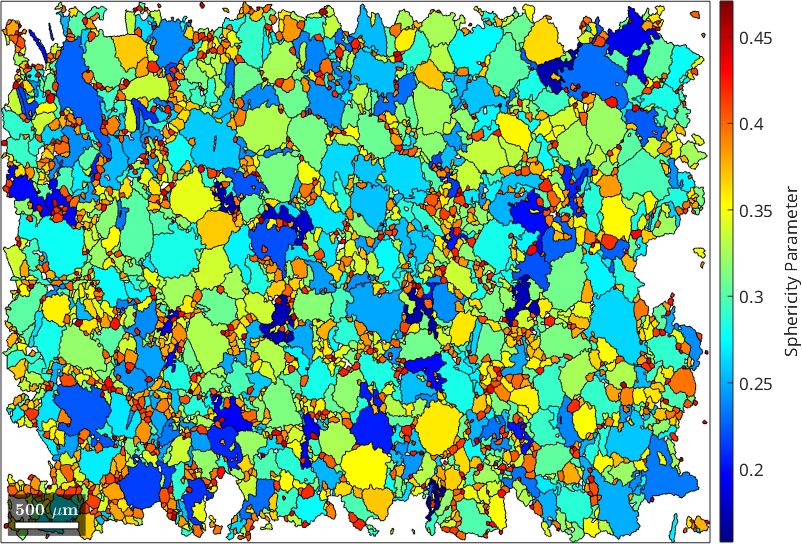## Influence of EBSD step size on sphericity parameter

Next we investigate how step size influences grain boundary irregularity measurements. To do this, we can artificially increase the step size of the EBSD data to from 10 up to 100 ÿm. Then, we choose one representative grain (one with a large number of pixels) and see how the sphericity parameter changes as the EBSD step size increases.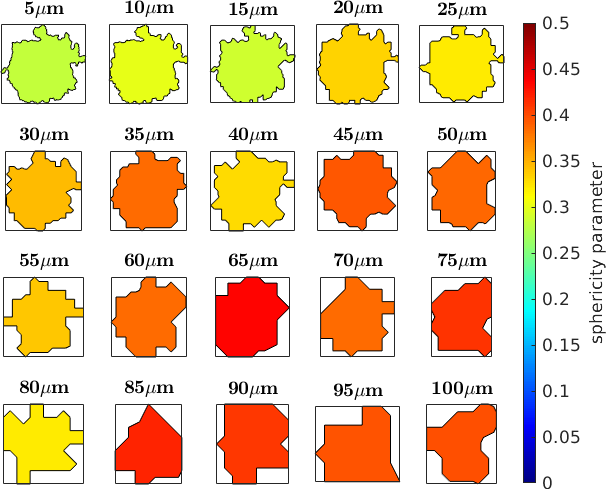Now we are able to plot the sphericity parameter as a function of the step size.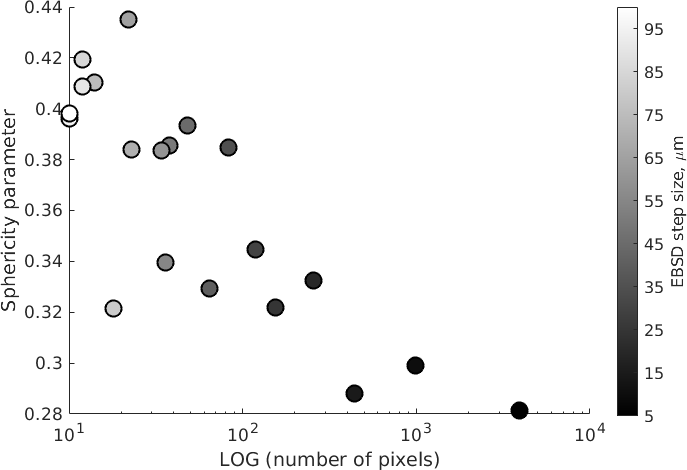## Influence of grain boundary smoothness on sphericity parameter

Due to the square shape of pixels (prescribed by Oxford Instruments software), boundary elements lie either vertically or horizontally within the plane of analysis. Grains containing fewer pixels (i.e., smaller grains in maps with a fixed step size) appear more pixelated than grains containing numerous pixels. MTEX allows us to reduce artificial pixelation of grain boundary elements by applying the function smooth, which enhances the overall grain boundary smoothness by interpolating the coordinates of grain boundary elements while triple junction points remain locked or unlocked. In this study, we choose to lock the triple junction during grain boundary smoothening.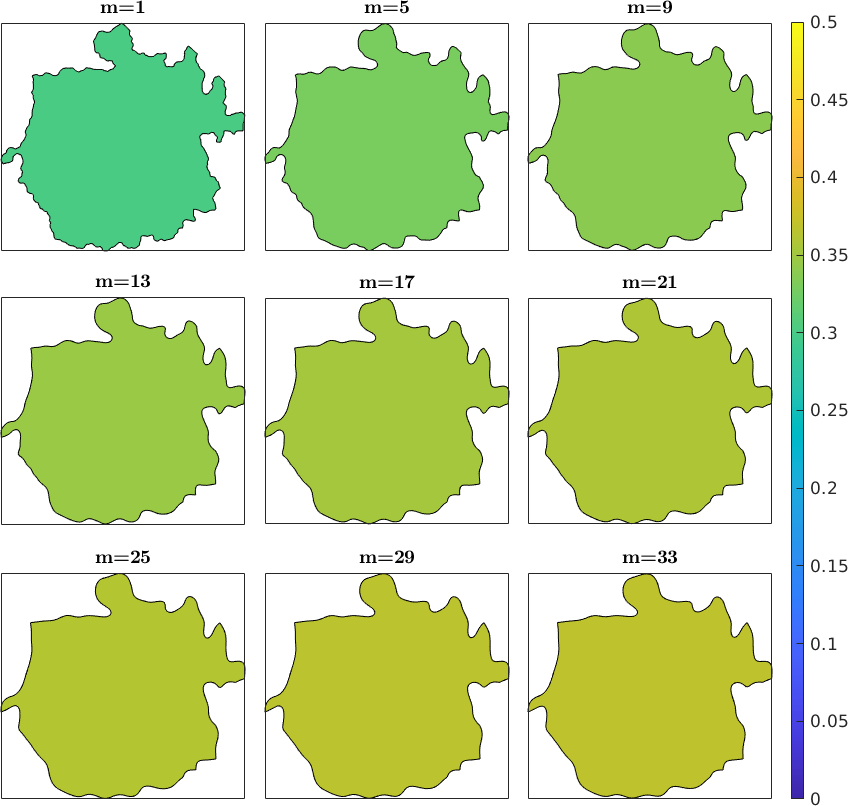Finally we plot the sphericity as a function of the smoothening parameter